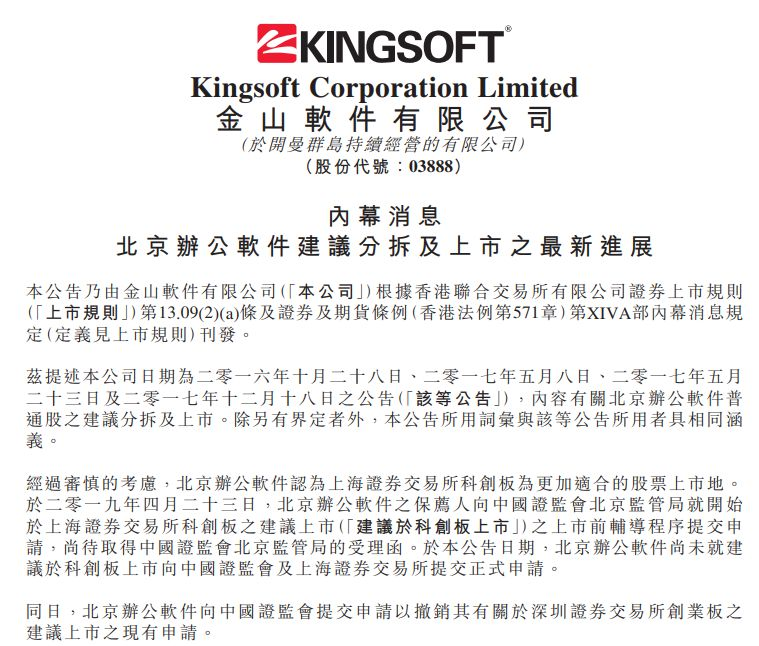浏览次数：4873发布时间：2020-01-09 10:31:571·小数量，一般在三位数以下;

2·分散性，经常有“跳价位”挂单;

3·整数性，即喜欢在股价的整数位挂出买卖单，比如9元、9.5元、9.8元和10元等

1、递增压迫式

2、递减压迫式

1、对称性蜂窝式挂单

2、非对称性蜂窝式挂单

tj1:=ref(cross(ma(c,5),c),1) and cross(c,ma(c,5));

tj2:=ref(cross(ma(c,10),c),1) and cross(c,ma(c,10));

tj3:=ref(cross(ma(c,20),c),1) and cross(c,ma(c,20));

tj4:=tj1 or tj2 or tj3;

tj5:=ref(c,2)/ref(c,1)>=1.045 and ref(c,1)1.09 and c=h and tj4;

a:=(3*c+l+o+h)/6;

x:=(20*a+19*ref(a,1)+18*ref(a,2)+17*ref(a,3)+16*ref(a,4)+15*ref(a,5)+

14*ref(a,6)+13*ref(a,7)+12*ref(a,8)+11*ref(a,9)+10*ref(a,10)+9*ref(a,11)+8*ref(a,12)

+7*ref(a,13)+6*ref(a,14)+5*ref(a,15)+4*ref(a,16)+3*ref(a,17)+2*ref(a,18)+

ref(a,20))/210;

kka:=ema(c,5);

kkb:=ema(kka,8);

kkc:=ema(kkb,13);

kaaa3:=ref(c,1)中线;

kbbb3:=c/ref(c,1)>1+0.01*9.00;

kccc3:=中线/长线1<1+0.01*20.00;

(以上内容仅供参考，不构成操作建议。如自行操作，注意仓位控制和风险自负。)